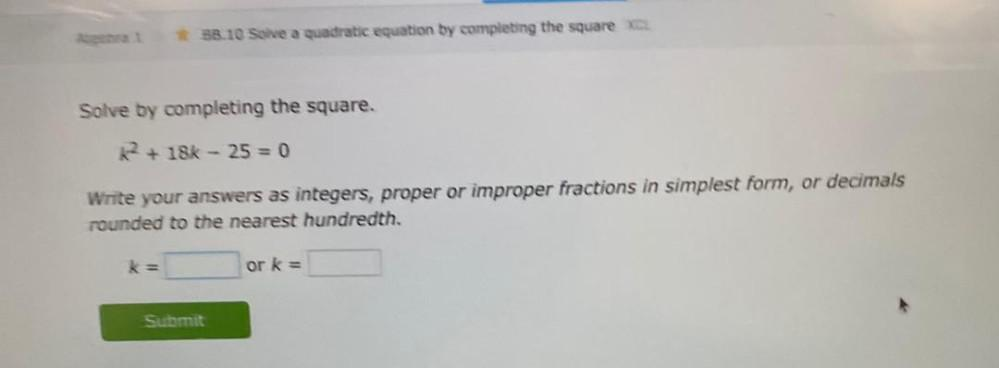Question:

# Solve by completing the square. k^2 + 18k - 25 = 0 Write your answers as integers, proper or improper fractions in simplest form, or decimals rounded to the nearest hundredth,Solve by completing the square. k^2 + 18k - 25 = 0 Write your answers as integers, proper or improper fractions in simplest form, or decimals rounded to the nearest hundredth,